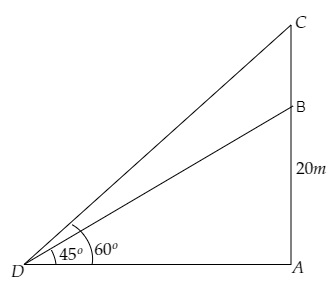# From a point on the ground, the angles of elevation of the bottom and the top of a transmission tower fixed at the top of a $20\ m$ high building are $45^o$ and $60^o$ respectively. Find the height of the tower.

Given:

From a point on the ground the angles of elevation of the bottom and top of a transmission tower fixed at the top of $20 \mathrm{~m}$ high building are $45^{\circ}$ and $60^{\circ}$ respectively.

To do:

We have to find the height of the transmission tower.

Solution:Let $AB$ be the high building and $BC$ be the length of the transmission tower.

Let point $D$ be the point of observation.

From the figure,

$\mathrm{AB}=20 \mathrm{~m}, \angle \mathrm{BDA}=45^{\circ}, \angle \mathrm{CDA}=60^{\circ}$

Let the height of the transmission tower be $\mathrm{BC}=h \mathrm{~m}$ and the distance between the point of observation and the foot of the building be $\mathrm{AD}=x \mathrm{~m}$.

This implies,

$\mathrm{AC}=20+h \mathrm{~m}$

We know that,

$\tan \theta=\frac{\text { Opposite }}{\text { Adjacent }}$

$=\frac{\text { AB }}{DA}$

$\Rightarrow \tan 45^{\circ}=\frac{20}{x}$

$\Rightarrow 1(x)=20$

$\Rightarrow x=20 \mathrm{~m}$

Similarly,

$\tan \theta=\frac{\text { Opposite }}{\text { Adjacent }}$

$=\frac{\text { CA }}{DA}$

$\Rightarrow \tan 60^{\circ}=\frac{20+h}{x}$

$\Rightarrow \sqrt3=\frac{20+h}{20}$

$\Rightarrow 20+h=20\sqrt3 \mathrm{~m}$

$\Rightarrow h=20(\sqrt3-1) \mathrm{~m}$

Therefore, the height of the transmission tower is $20(\sqrt3-1) \mathrm{~m}$.

Updated on: 10-Oct-2022

49 Views